单线程、多线程和协程的爬虫性能对比豆瓣深圳影讯爬虫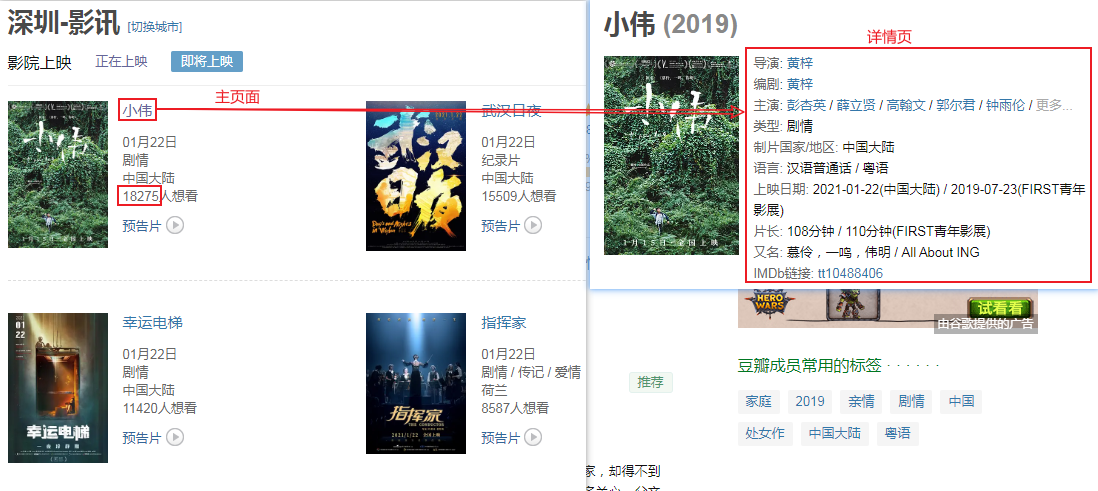爬取测试

import requestsfrom lxml import etreeimport pandas as pdimport re
main_url = "https://movie.douban.com/cinema/later/shenzhen/"headers = { "Accept-Encoding": "Gzip", "User-Agent": "Mozilla/5.0 (Windows NT 6.1; WOW64) AppleWebKit/537.36 (KHTML, like Gecko) Chrome/78.0.3904.108 Safari/537.36"}r = requests.get(main_url, headers=headers)r

<Response >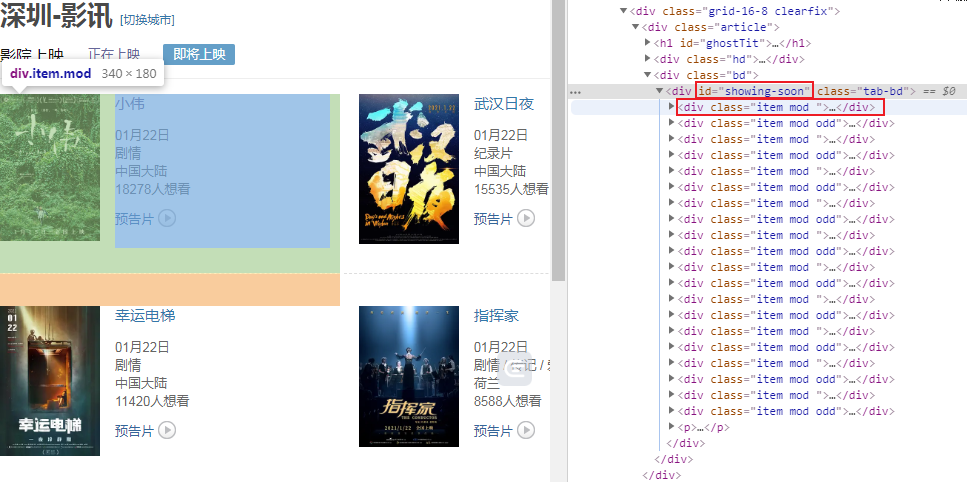html = etree.HTML(r.text)all_movies = html.xpath("//div[@id='showing-soon']/div")result = []for e in all_movies:    #  imgurl, = e.xpath(".//img/@src")    name, = e.xpath(".//div[@class='intro']/h3/a/text()")    url, = e.xpath(".//div[@class='intro']/h3/a/@href")    # date, movie_type, pos = e.xpath(".//div[@class='intro']/ul/li[@class='dt']/text()")    like_num, = e.xpath(        ".//div[@class='intro']/ul/li[@class='dt last']/span/text()")    result.append((name, int(like_num[:like_num.find("人")]), url))main_df = pd.DataFrame(result, columns=["影名", "想看人数", "url"])main_df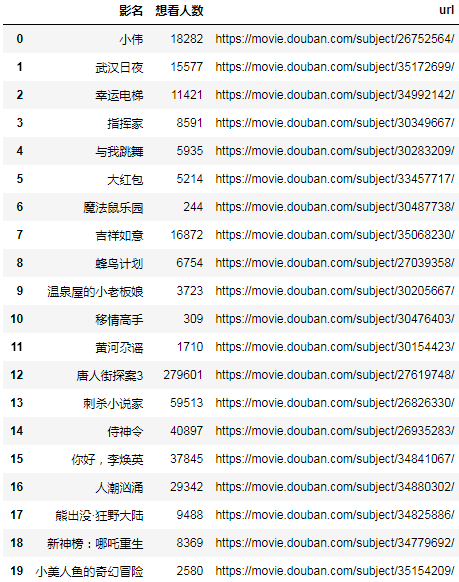url = main_df.at[17, "url"]url

'https://movie.douban.com/subject/34825886/'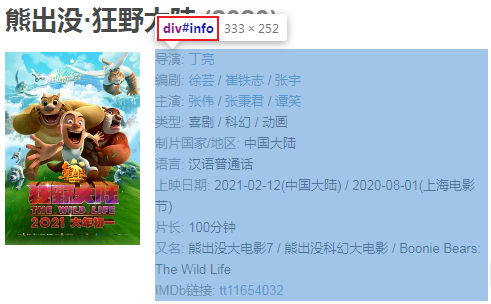['\n        ', '导演', ': ', '丁亮', '\n        ', '编剧', ': ', '徐芸', ' / ', '崔铁志', ' / ', '张宇', '\n        ', '主演', ': ', '张伟', ' / ', '张秉君', ' / ', '谭笑', '\n        ', '类型:', ' ', '喜剧', ' / ', '科幻', ' / ', '动画', '\n        \n        ', '制片国家/地区:', ' 中国大陆', '\n        ', '语言:', ' 汉语普通话', '\n        ', '上映日期:', ' ', '2021-02-12(中国大陆)', ' / ', '2020-08-01(上海电影节)', '\n        ', '片长:', ' ', '100分钟', '\n        ', '又名:', ' 熊出没大电影7 / 熊出没科幻大电影 / Boonie Bears: The Wild Life', '\n        ', 'IMDb链接:', ' ', 'tt11654032', '\n\n']

movie_info_txt = "".join(movie_infos)print(movie_info_txt)

row = {}for line in re.split("[\n ]*\n[\n ]*", movie_info_txt):    line = line.strip()    arr = line.split(": ", maxsplit=1)    if len(arr) != 2:        continue    k, v = arr    row[k] = vrow

{'导演': '丁亮', '编剧': '徐芸 / 崔铁志 / 张宇', '主演': '张伟 / 张秉君 / 谭笑', '类型': '喜剧 / 科幻 / 动画', '制片国家/地区': '中国大陆', '语言': '汉语普通话', '上映日期': '2021-02-12(中国大陆) / 2020-08-01(上海电影节)', '片长': '100分钟', '又名': '熊出没大电影7 / 熊出没科幻大电影 / Boonie Bears: The Wild Life', 'IMDb链接': 'tt11654032'}

单线程爬虫

import requestsfrom lxml import etreeimport pandas as pdimport re
main_url = "https://movie.douban.com/cinema/later/shenzhen/"headers = { "Accept-Encoding": "Gzip", "User-Agent": "Mozilla/5.0 (Windows NT 6.1; WOW64) AppleWebKit/537.36 (KHTML, like Gecko) Chrome/78.0.3904.108 Safari/537.36"}r = requests.get(main_url, headers=headers)html = etree.HTML(r.text)all_movies = html.xpath("//div[@id='showing-soon']/div")result = []for e in all_movies: imgurl, = e.xpath(".//img/@src") name, = e.xpath(".//div[@class='intro']/h3/a/text()") url, = e.xpath(".//div[@class='intro']/h3/a/@href") print(url)# date, movie_type, pos = e.xpath(".//div[@class='intro']/ul/li[@class='dt']/text()") like_num, = e.xpath( ".//div[@class='intro']/ul/li[@class='dt last']/span/text()") r = requests.get(url, headers=headers) html = etree.HTML(r.text) row = {} row["电影名称"] = name for line in re.split("[\n ]*\n[\n ]*", "".join(html.xpath("//div[@id='info']//text()")).strip()): line = line.strip() arr = line.split(": ", maxsplit=1) if len(arr) != 2: continue k, v = arr row[k] = v row["想看人数"] = int(like_num[:like_num.find("人")])# row["url"] = url# row["图片地址"] = imgurl# print(row) result.append(row)df = pd.DataFrame(result)df.sort_values("想看人数", ascending=False, inplace=True)df.to_csv("shenzhen_movie.csv", index=False)

https://movie.douban.com/subject/26752564/https://movie.douban.com/subject/35172699/https://movie.douban.com/subject/34992142/https://movie.douban.com/subject/30349667/https://movie.douban.com/subject/30283209/https://movie.douban.com/subject/33457717/https://movie.douban.com/subject/30487738/https://movie.douban.com/subject/35068230/https://movie.douban.com/subject/27039358/https://movie.douban.com/subject/30205667/https://movie.douban.com/subject/30476403/https://movie.douban.com/subject/30154423/https://movie.douban.com/subject/27619748/https://movie.douban.com/subject/26826330/https://movie.douban.com/subject/26935283/https://movie.douban.com/subject/34841067/https://movie.douban.com/subject/34880302/https://movie.douban.com/subject/34825886/https://movie.douban.com/subject/34779692/https://movie.douban.com/subject/35154209/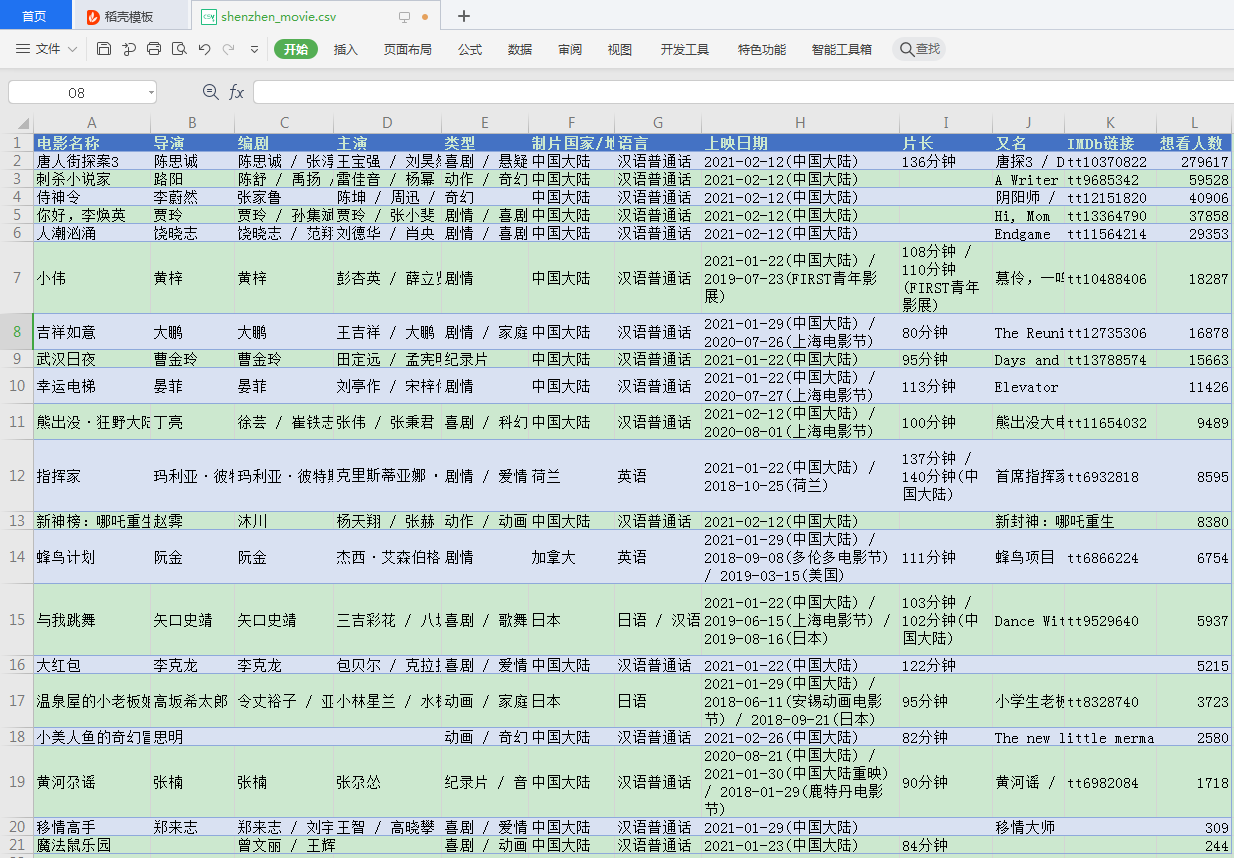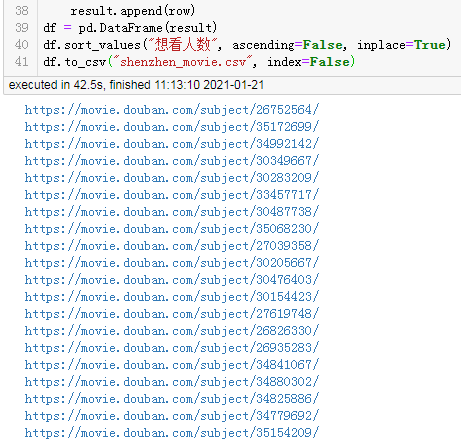42.5 秒。

多线程爬虫

import requestsfrom lxml import etreeimport pandas as pdimport refrom concurrent.futures import ThreadPoolExecutor, wait, ALL_COMPLETED

def fetch_content(url): print(url) headers = { "Accept-Encoding": "Gzip", # 使用gzip压缩传输数据让访问更快 "User-Agent": "Mozilla/5.0 (Windows NT 6.1; WOW64) AppleWebKit/537.36 (KHTML, like Gecko) Chrome/78.0.3904.108 Safari/537.36" } r = requests.get(url, headers=headers) return r.text

url = "https://movie.douban.com/cinema/later/shenzhen/"init_page = fetch_content(url)html = etree.HTML(init_page)all_movies = html.xpath("//div[@id='showing-soon']/div")result = []for e in all_movies:# imgurl, = e.xpath(".//img/@src") name, = e.xpath(".//div[@class='intro']/h3/a/text()") url, = e.xpath(".//div[@class='intro']/h3/a/@href")# date, movie_type, pos = e.xpath(".//div[@class='intro']/ul/li[@class='dt']/text()") like_num, = e.xpath( ".//div[@class='intro']/ul/li[@class='dt last']/span/text()") result.append((name, int(like_num[:like_num.find("人")]), url))main_df = pd.DataFrame(result, columns=["影名", "想看人数", "url"])
max_workers = main_df.shapewith ThreadPoolExecutor(max_workers=max_workers) as executor: future_tasks = [executor.submit(fetch_content, url) for url in main_df.url] wait(future_tasks, return_when=ALL_COMPLETED) pages = [future.result() for future in future_tasks]
result = []for url, html_text in zip(main_df.url, pages): html = etree.HTML(html_text) row = {} for line in re.split("[\n ]*\n[\n ]*", "".join(html.xpath("//div[@id='info']//text()")).strip()): line = line.strip() arr = line.split(": ", maxsplit=1) if len(arr) != 2: continue k, v = arr row[k] = v row["url"] = url result.append(row)detail_df = pd.DataFrame(result)df = main_df.merge(detail_df, on="url")df.drop(columns=["url"], inplace=True)df.sort_values("想看人数", ascending=False, inplace=True)df.to_csv("shenzhen_movie2.csv", index=False)df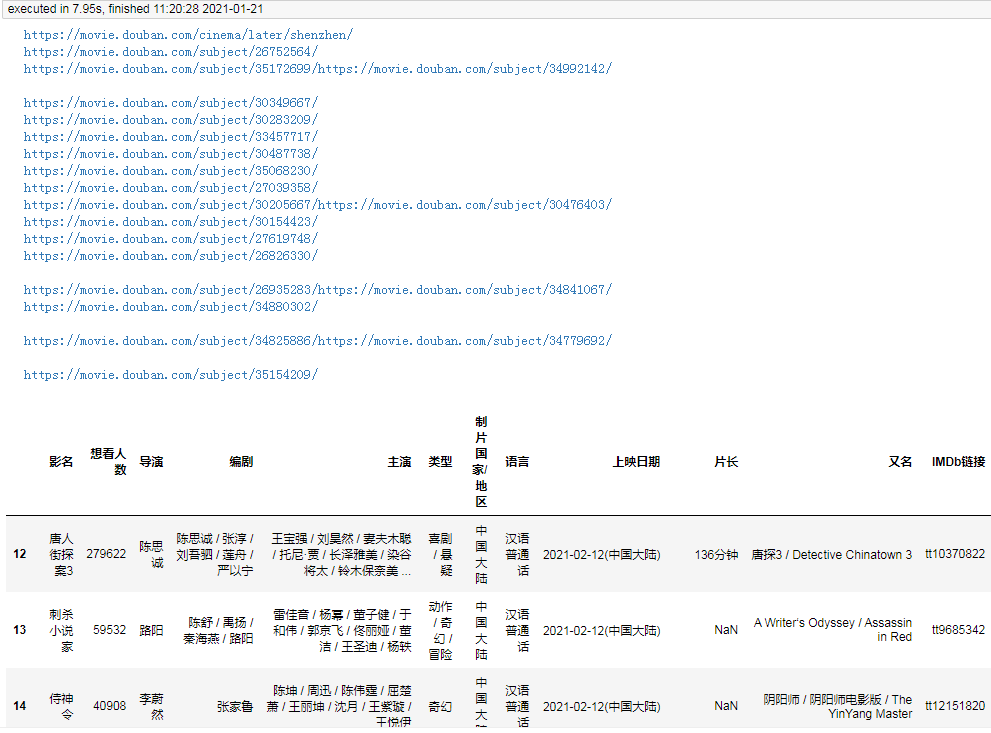异步协程爬虫

import nest_asyncionest_asyncio.apply()

import aiohttpfrom lxml import etreeimport pandas as pdimport reimport asyncioimport nest_asyncionest_asyncio.apply()

async def fetch_content(url): print(url) header = { "Accept-Encoding": "Gzip", # 使用gzip压缩传输数据让访问更快 "User-Agent": "Mozilla/5.0 (Windows NT 6.1; WOW64) AppleWebKit/537.36 (KHTML, like Gecko) Chrome/78.0.3904.108 Safari/537.36" } async with aiohttp.ClientSession( headers=header, connector=aiohttp.TCPConnector(ssl=False) ) as session: async with session.get(url) as response: return await response.text()

async def main(): url = "https://movie.douban.com/cinema/later/shenzhen/" init_page = await fetch_content(url) html = etree.HTML(init_page) all_movies = html.xpath("//div[@id='showing-soon']/div") result = [] for e in all_movies: # imgurl, = e.xpath(".//img/@src") name, = e.xpath(".//div[@class='intro']/h3/a/text()") url, = e.xpath(".//div[@class='intro']/h3/a/@href") # date, movie_type, pos = e.xpath(".//div[@class='intro']/ul/li[@class='dt']/text()") like_num, = e.xpath( ".//div[@class='intro']/ul/li[@class='dt last']/span/text()") result.append((name, int(like_num[:like_num.find("人")]), url)) main_df = pd.DataFrame(result, columns=["影名", "想看人数", "url"])
result = [] for url, html_text in zip(main_df.url, pages): html = etree.HTML(html_text) row = {} for line in re.split("[\n ]*\n[\n ]*", "".join(html.xpath("//div[@id='info']//text()")).strip()): line = line.strip() arr = line.split(": ", maxsplit=1) if len(arr) != 2: continue k, v = arr row[k] = v row["url"] = url result.append(row) detail_df = pd.DataFrame(result) df = main_df.merge(detail_df, on="url") df.drop(columns=["url"], inplace=True) df.sort_values("想看人数", ascending=False, inplace=True) return df
df = asyncio.run(main())df.to_csv("shenzhen_movie3.csv", index=False)df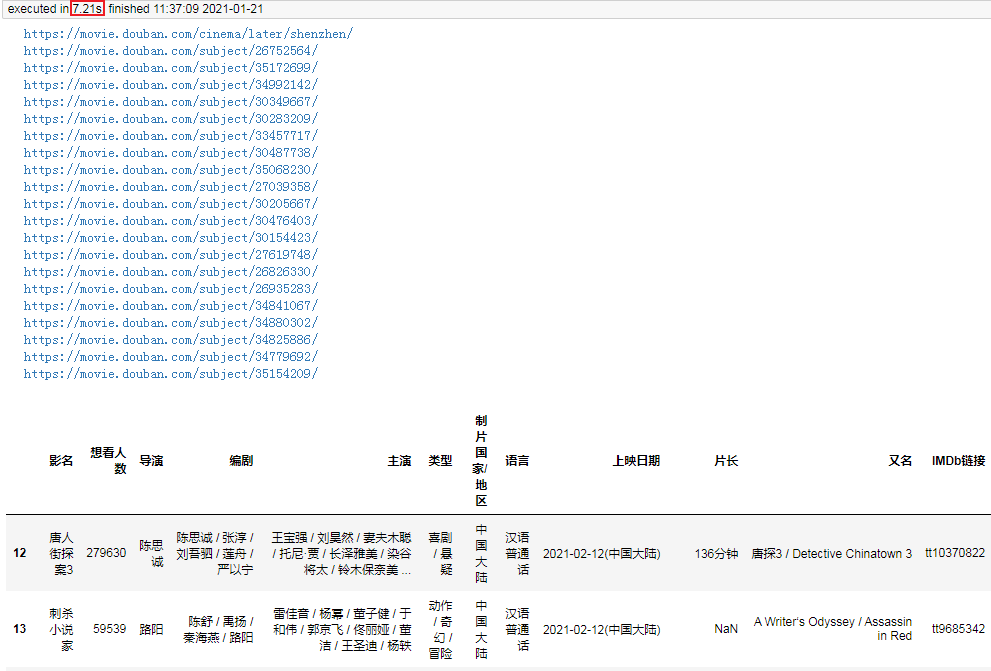asyncio 知识点补充

asyncio.run(main())表示拿到 event loop，运行输入的 coro(main()函数)，直到结束后关闭这个 event loop。

loop = asyncio.get_event_loop()try:    loop.run_until_complete(coro)finally:    loop.close()

asyncio.create_task(coro)，表示对输入的协程 coro 创建一个任务，安排它的执行，并返回此任务对象。这个函数也只能在 Python 3.7 以上版本使用，对于 3.7 以下版本可以用asyncio.ensure_future(coro)替代。

彩蛋：xpath+pandas 解析表格并提取 url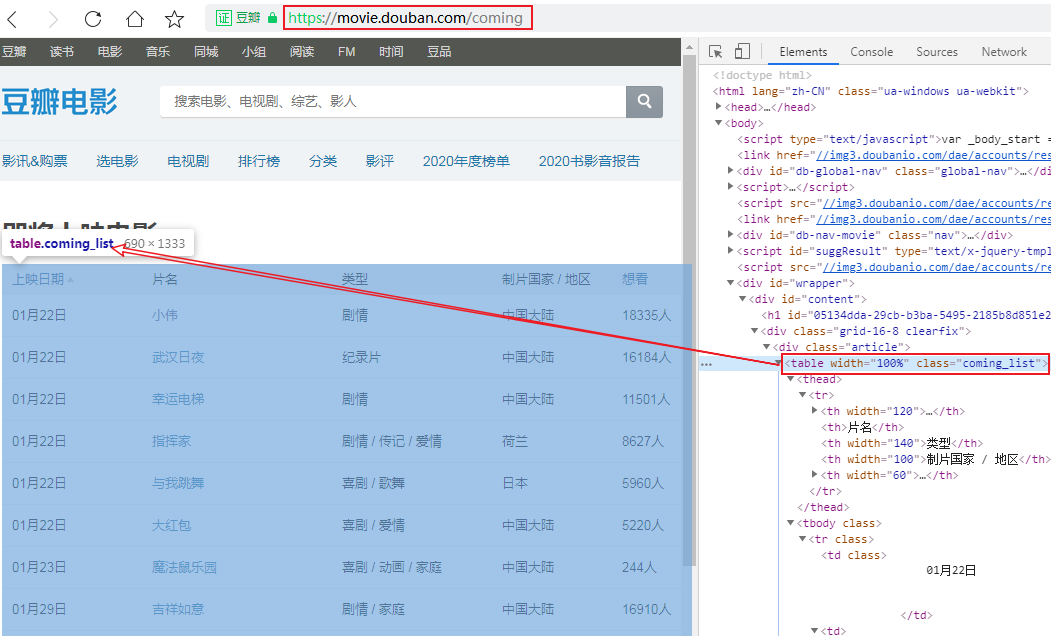import pandas as pdimport requestsfrom lxml import etree
headers = { "Accept-Encoding": "Gzip", "User-Agent": "Mozilla/5.0 (Windows NT 6.1; WOW64) AppleWebKit/537.36 (KHTML, like Gecko) Chrome/78.0.3904.108 Safari/537.36"}r = requests.get("https://movie.douban.com/coming", headers=headers)html = etree.HTML(r.text)table_tag = html.xpath("//table")df, = pd.read_html(etree.tostring(table_tag))urls = table_tag.xpath(".//td/a/@href")df["url"] = urlsdf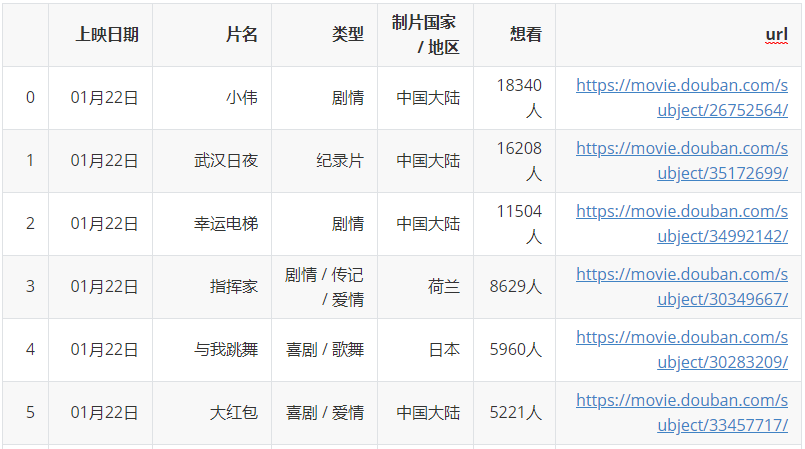结语评论